# Offset Formula in Microsoft Excel

One of the rows

- How many rows you want to move the starting point and both of these can be positive, negative or zero.

Height and Width: - This is the size of the range you want to return.

Let’s take an example to understand the Offset function how works in excel.

We have data in range A1:D10. Column A contains Product Code, Column B contains Quantity, column C contains per product cost and column D contains Total cost. We want to return the value of cell C5 in cell E2.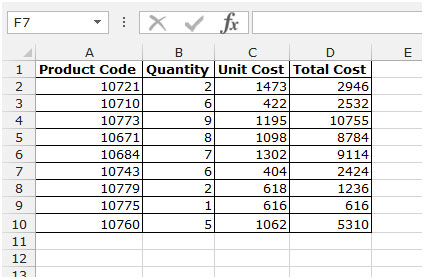• Select the cell E2 and write the formula.
• =OFFSET(A1,4,2,1,1) press enter.
• The function will return the value of cell C5.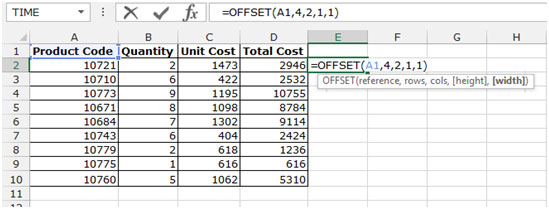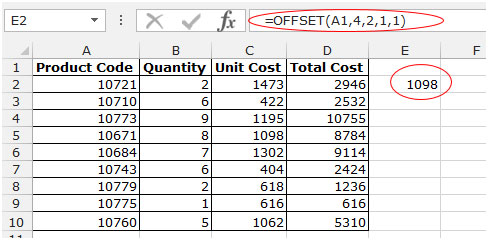Use the Offset function along with Sum function

• Select the cell E3 and write the formula.
• =SUM(OFFSET(A1,5,1,1,2))press enter.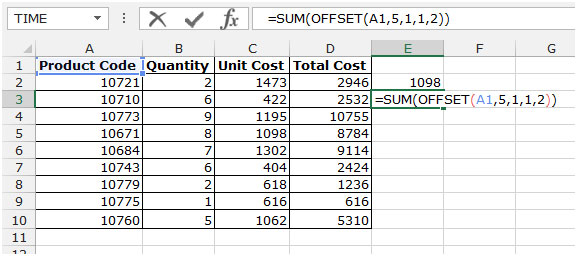• The function will return the sum of Cell B6+C6 = 1309.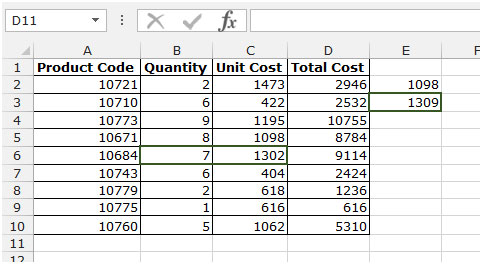Note: Before you insert the offset function (without having to calculate totals) to return to a range, select a category of the same size if you specify rows a cell or range of cells above or to the left is the number of columns that want to come back, enter a negative number.

This is all about the Offset function and its uses in Microsoft Excel 2010 and 2013.

Terms and Conditions of use

The applications/code on this site are distributed as is and without warranties or liability. In no event shall the owner of the copyrights, or the authors of the applications/code be liable for any loss of profit, any problems or any damage resulting from the use or evaluation of the applications/code.